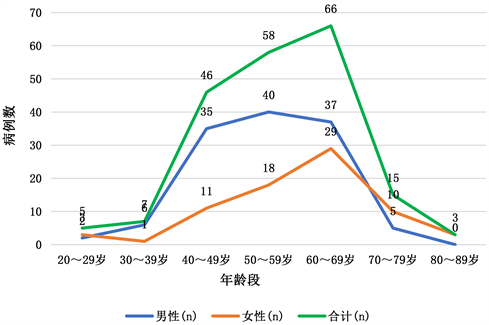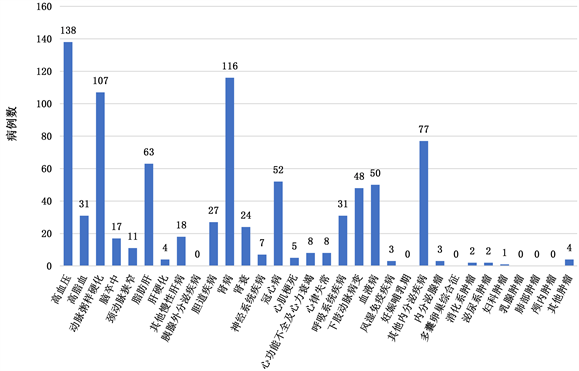期刊菜单

Analysis of Diabetes with Chronic Complications and Its Risk Factors

Abstract: 200 cases of type II diabetes with chronic complications and its risk factors were studied in this paper. First of all, descriptive statistical analysis was conducted on the age and sex ratio of patients, as well as the composition of concurrent diseases. It was found that the patients mainly concentrated in the age group of 40~69 years old, and the sex composition of different age groups was different; in the situation of diabetic patients with retinopathy, there are a large proportion of other concurrent diseases, such as hypertension, nephropathy and atherosclerosis. Then, based on Logistic stepwise regression, random forest algorithm and test of association, the risk factors of hypertension in diabetic patients were comprehensively analyzed. The results showed that: AGE, BP_HIGH, SCR, SUA, BMI, and BU are the key risk factors.

1. 引言

2. 数据来源及预处理

2.1. 数据来源

2.2. 数据预处理

2.2.1. 缺失数据处理

2.2.2. 数据标准化

3. 并发症以及危险因素分析

3.1. 描述性分析

3.1.1. 200例II型糖尿病患者的年龄及性别构成比Table 1. Age and sex composition of 200 cases with type II diabetesFigure 1. Age group composition of patients

3.1.2. 200例II型糖尿病患者慢性并发症的疾病构成Figure 2. Constitution of diabetic complicationsTable 2. Composition of complications

3.2. 并发症危险因素的Logistic回归和随机森林分析

3.2.1. Logistic回归分析

Logistic回归是一种非线性回归模型，实际上是一种分类模型，并常用于二分类问题研究中。Logistic回归可以表示为：

$\mathrm{ln}\frac{p}{1-p}={\beta }_{0}+{\beta }_{1}{x}_{1}+{\beta }_{2}{x}_{2}+\cdots +{\beta }_{p}{\beta }_{p}+\cdots$Table 3. Logistic stepwise regression: risk factors

3.2.2. 随机森林(Random Forest)Table 4. Random forest: Risk factors

3.3. 相关性检验

${X}_{w}^{2}={\left(\mathrm{log}\stackrel{^}{OR}\right)}^{2}{\left(\frac{1}{{\stackrel{^}{e}}_{1}}+\frac{1}{{\stackrel{^}{e}}_{2}}+\frac{1}{{\stackrel{^}{f}}_{1}}+\frac{1}{{\stackrel{^}{f}}_{2}}\right)}^{-1}=\frac{{\left(\mathrm{log}\stackrel{^}{OR}\right)}^{2}{r}_{1}{r}_{2}{m}_{1}{m}_{2}}{{r}^{3}}$

${X}_{p}^{2}=\frac{{\left({a}_{1}-{\stackrel{^}{e}}_{1}\right)}^{2}}{{\stackrel{^}{e}}_{1}}+\frac{{\left({a}_{2}-{\stackrel{^}{e}}_{2}\right)}^{2}}{{\stackrel{^}{e}}_{2}}+\frac{{\left({b}_{1}-{\stackrel{^}{f}}_{1}\right)}^{2}}{{\stackrel{^}{f}}_{1}}+\frac{{\left({b}_{2}-{\stackrel{^}{f}}_{2}\right)}^{2}}{{\stackrel{^}{f}}_{2}}$

${X}_{lr}^{2}=2\left[{a}_{1}\mathrm{log}\left(\frac{{a}_{1}}{{\stackrel{^}{e}}_{1}}\right)+{a}_{1}\mathrm{log}\left(\frac{{a}_{2}}{{\stackrel{^}{e}}_{2}}\right)+{b}_{1}\mathrm{log}\left(\frac{{b}_{1}}{{\stackrel{^}{f}}_{1}}\right)+{b}_{2}\mathrm{log}\left(\frac{{b}_{2}}{{\stackrel{^}{f}}_{2}}\right)\right]$Table 5. Age-hypertension observation (expectation) counts contingency table

${X}_{p}^{2}=\frac{{\left(134-129.72\right)}^{2}}{129.72}+\frac{{\left(54-58.28\right)}^{2}}{58.28}+\frac{{\left(4-8.28\right)}^{2}}{8.28}+\frac{{\left(8-3.72\right)}^{2}}{3.72}=7.49\text{\hspace{0.17em}}\left(p=0.0062\right)$

${X}_{w}^{2}={\left(\mathrm{log}4.96\right)}^{2}\left(\frac{1}{129.72}+\frac{1}{58.28}+\frac{1}{8.28}+\frac{1}{3.72}\right)=6.12\text{\hspace{0.17em}}\left(p=0.0134\right)$

${X}_{lr}^{2}=2×\left[134\mathrm{log}\frac{134}{129.72}+54\mathrm{log}\frac{54}{58.28}+4\mathrm{log}\frac{4}{8.28}+8\mathrm{log}\frac{8}{3.72}\right]=6.92\text{\hspace{0.17em}}\left(p=0.0085\right)$Table 6. Pearson, Wald, LR Test Statistic and p value of 7 risk factors

4. 总结与讨论

NOTES

*通讯作者。

  楼雪勇. 2 型糖尿病患者慢性并发症患病率及危险因素分析[J]. 中国现代医生, 2011, 49(4): 120-121.  谢利平, 王国洪. 1436例2型糖尿病患者慢性并发症合并率及相关因素的统计分析[J]. 中国病案, 2015, 16(8): 92-94.  邹飒枫, 乔晶. 4158例高血压合并糖尿病病人现况及并发症分析[J]. 中华疾病控制杂志, 2010, 14(11): 1124-1125.  李纯净, 丁雪, 李芸, 等. 删失数据下部分线性模型对糖尿病并发症的统计分析[J]. 长春工业大学学报, 2020, 41(2): 105-111+209.  彭浩, 丁建松, 彭颖, 等. 女性人群血清尿酸水平与高血压及高血压前期的关系[J]. 中华高血压杂志, 2011, 19(3): 236-239.  吴云涛, 吴寿岭, 李云, 等. 血清尿酸对高血压前期人群血压转归的影响[J]. 中华高血压杂志, 2010, 18(6): 545-549.  张红叶, 李莹, 陶寿淇, 等. 血清尿酸与四年后血压变化及高血压发病的关系[J]. 高血压杂志, 2001(2): 72-75.  中国人民解放军总医院. 共享杯版_糖尿病并发症预警数据集[DB/OL] https://www.ncmi.cn/phda/dataDetails.do?id=CSTR:A0006.11.A0005.202006.001018, 2021-06-28.  Newman, S.C. (2001) Biostatistical Methods in Epidemiology. 2nd Edition, John Wiley & Sons, Inc., New York, 94-98.